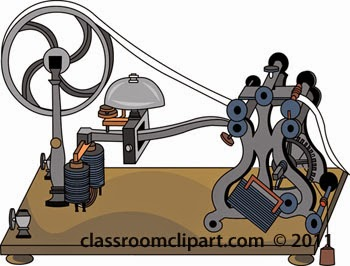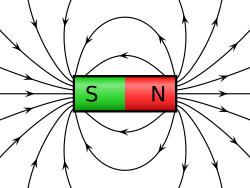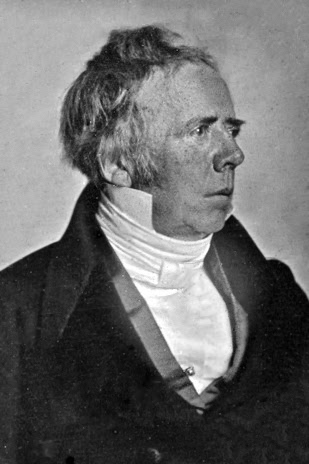## Thursday, 10 April 2014

### CBSE Class 10 - Science - CH13 - Magnetic Effects of Electric Current

Magnetic Effects of Electric Current

Q & AMorse Telegraph which uses Magnetic effect of Currentimage credits: classroomclipart.com
Q1: What is meant by Magnetic effect of Electric current?

Answer: It means that a current flowing in a wire produces a magnetic field around it. In other words, electric current can produce magnetism.

Q2: What is a magnet?

Answer: A magnet is an object which attracts pieces of iron, steel, nickel and cobalt.

Q3: What is a Bar magnet?

Answer: A bar magnet is a long rectangular bar of uniform cross-section which attracts pieces of iron, steel nickel and cobalt.

Like poles repel, while unlike poles of magnets attract each other.

Q4: Define Magnetic field:

Answer: The space surrounding a magnet in which magnetic force is exerted is called a magnetic field. The magnetic field has both, magnitude and direction.

Q5: What is the direction of magnetic field?

Answer: The direction of magnetic field at a point is the direction of the resultant force acting on a hypothetical north pole placed at that point. The north end of the needle of a compass indicates the direction of magnetic field at a point where it is placed.

Q6: Why does a compass needle get deflected when brought near a bar magnet?

Answer: It is due to the magnetic force exerted by the magnet. A compass needle is in fact, a small bar magnet. It has been found by experiments that like poles repel each other whereas unlike poles attract each other. Hence, the deflection.

A compass needle gets deflected when brought near a bar magnet because the bar magnet exerts a magnetic force on the compass needle, which is itself a tiny pivoted magnet (free to move in the horizontal plane.)

Q7: What are magnetic field lines or magnetic lines of force?

Answer: The magnetic field lines are the lines drawn in a magnetic field along which a north magnetic pole would move. The direction of a magnetic field at any point gives the direction of the magnetic force on a north pole placed at that point. Since the direction of magnetic field line is the direction of force, on north pole, so the magnetic field lines always begin from the North pole of a magnet and end on the South pole of the magnet. Inside, the magnet however, the direction of magnetic field line is from the south pole to the north pole of the magnet. Thus, the magnetic field lines are closed curves.Magnetic Lines of Force image credits: wikipedia
The relative strength of the magnetic field is shown by the degree of closeness of the field lines, where the field lines are closest together, the magnetic field is the strongest. For example, the field lines are closest together at the two poles, so the magnetic field is the strongest at the poles.

Q8: List the properties of the magnetic field lines.

Answer: Properties of the magnetic field lines:

. The magnetic field lines originate from the north pole of a magnet and end at its south pole.

. The field lines come closer to one another near the poles of a magnet but they are widely separated at other places. Where magnetic field lines are closer together, it indicates a stronger magnetic field. On the other hand, when magnetic field lines are widely separated, then it indicates a weak magnetic field.

. No two field lines cross each other. This is due to the fact that the resultant force on a north pole at any point can be only in one direction. But if the two field lines cross each other, then the resultant force on a north pole placed at the point of intersection will be along two directions, which is not possible.

Q9: Why do the iron filings placed near a bar magnet arrange in a particular pattern? What does this pattern demonstrate?

Answer: The magnet exerts a magnetic field all around it. The iron filings experience the force of magnetic field of the magnet. This force makes the iron filings to arrange themselves in a particular pattern. In fact, under the influence of the magnetic field do the magnet, the iron filings behave like tiny magnets and align themselves along the direction of magnetic field lines. The lines along which the iron filings align themselves represent magnetic field lines.

Q10: Write a short note on the magnetic field of the earth.

Answer: A freely suspended magnet always points in the north-south direction even in the absence of any other magnet. This is due to the fact that the earth itself behave like a magnet which causes a freely suspended magnet (or magnetic needle) to point always in north and south direction. The shape of the earth’s magnetic field resembles that of an imaginary bar magnet of length one fifth of earth’s diameter buried at its center.

The south pole of earth’s magnet is in the geographical north because it attracts the north pole of the suspended magnet. Similarly, the north pole of earth’s magnet is in the geographical south.

The axis of earth's magnet and the geographical axis do not coincide with each other. The axis of earth’s magnetic field is inclined at an angle of about 15° degree with the geographical axis. Due to this a freely suspended magnet makes angle of about 15° degree with the geographical axis and points only approximately in the north-south directions at a place. (or A freely suspended magnet does not show exact geographical north and south because the magnetic axis and geographical axis of the earth do not coincide. The earth’s magnetism is due to the magnetic effect of current which is flowing in the liquid-core at the center of the earth.Hans_Christian_Ørsted credits: wikipedia

Q11: Who discovered Magnetic effect of current?

Answer: Oersted discovered the magnetic effect of current in 1920.

Q12: What happens when an electric current passes through a metallic conductor?

Answer: An electric current through a metallic conductor produces a magnetic field around it.

Q13: What is the importance of magnetic effect of current?

Answer: Important of magnetic effect of current – it gives rise to mechanical forces.Electric motor, electric generator, telephone and radio, all utilize the magnetic effect of current.

Q14: What do you mean by electromagnetism?

Answer: It means electricity produces magnetism. The deflection of compass needle by the current-carrying wire shows that an electric current produces a magnetic field which deflects the compass needle placed near the current carrying wire.

Q15: What happens to the compass needle if the direction of electric current is reversed?

Answer: If the direction of electric current is reversed the compass needle is deflected in the opposite direction. This shows that when we reverse the direction of electric current flowing in the wire, then the direction of magnetic field produced by it is also reversed.

Q16: What is the magnetic field pattern due to straight current carrying conductor?

Answer: Magnetic field(pattern) due to straight current – carrying conductor.

The magnetic field lines around a straight conductor (straight wire) carrying current are concentric circles whose centre lie on the wire. When current in the wire flows in the upward direction, then the field lines are in the anticlockwise direction. If the direction of current in the wire is reversed, the direction of magnetic field lines also gets reversed.

Q17: How does magnitude/stregth of magnetic field produced by a straight current carrying wire affected by current and the distance of a point from the wire?

Answer: The magnitude of magnetic field produced by a straight current carrying wire at a given point is:
1. Directly proportional to the current passing in the wire and
2. Inversely proportional to the distance of that point from the wire.

So, greater the current in the wire, stronger will be the magnetic field produced.

And greater the distance of a point from the magnetic field produced at that point. In fact, as we move
away from a current carrying straight wire, the concentric circles around it representing field lines,
become larger and larger indicating the decreasing strength of the magnetic field.

Q18: Name the rule that helps in finding the direction of magnetic field produced by current-carrying conductor (wire).

Answer: Maxswell’s right hand thumb rule.

Q19: Define  Maxswell’s right hand thumb rule.

Answer:  Imagine that you are holding a current-carrying straight conductor in your right hand such that the thumb points towards the direction of current. Then your finger will wrap around the conductor in the direction of the field lines of the magnetic field.

Q20: Describe Maxwell’s corkscrew rule.

Answer: Imagine, driving a corkscrew in the direction of current, then the direction in which we turn its handle is the direction of magnetic field (or magnetic field lines.)

Note: When electric current flows vertically upwards the direction of magnetic field produced is anticlockwise. On the other hand, when electric current flows vertically downwards then the direction of magnetic flow is clockwise.

Q21: A current through a horizontal power line flows in east to west direction. What is the direction of magnetic field at a point directly below it and at a point directly above it?
Answer: The current is in the east-west direction. Applying the right-hand thumb rule, we get that the direction of magnetic field at a point below the wire is from north to south and above the wire is from south to north.

Q22: What will be the magnetic field pattern due to a circular loop (or circular wire) carrying current?

Answer:  The magnetic effect of current increases, if a wire in the form of circular loop is used in place of a straight wire. When a current is passed through the circular loop of wire, a magnetic field is produced around it. The magnetic field lines are circular near the current-carrying loop. As we move away, the concentric circles representing magnetic field lines become bigger and bigger. At the centre of the circular loop, the magnetic field lines are straight. By applying right hand thumb rule, it is observed that each segment of circular loop carrying current produces magnetic field lines in the same direction within the loop. At the centre of the circular loop all the field lines are in the same direction and aid each other, due to which the strength of magnetic field increases.

Q23: How does the magnitude of the magnetic field produced by a current-carrying circular loop at its center related to the current flowing and the radius of the loop?

Answer: The magnitude of the magnetic field produced by a current-carrying circular loop at its center is:
• Directly proportional to the current passing through the circular loop and
• Inversely proportional to the radius of circular loop (or circular wire)

Q24: How can the strength of magnetic field produced by a current carrying circular coil be increased?

Answer: The strength of magnetic field produced by a current carrying circular coil can be increased:
1. By increasing the number of turns of wire in the coil,
2. By increasing the current flowing through the coil, and
3. By decreasing the radius of the coil.

Q25: What is a solenoid?

Answer: A coil of many circular turns of insulated copper wire wrapped closely in the shape of a cylinder is called a solenoid. The magnetic field produced by a current carrying solenoid is similar to the magnetic field produced by a bar magnet. The lines of magnetic field pass through the solenoid ad return to the other end. The magnetic field lines inside the solenoid are in the form of parallel straight lines. This indicates that the strength of magnetic field is the same at all the points inside the solenoid one end of the solenoid behaves a magnetic north pole, while the other behaves as the south pole.

The current in each turn of a solenoid flows in the same direction due to which the magnetic field produced by each turn adds up, giving a strong magnetic field inside the solenoid.

Q26: Name any use of solenoid?

Answer: A solenoid is used for making electromagnets.

Q27: On what factors does the strength of solenoid depend upon?

Answer: The strength of the magnetic field in a solenoid depends on:
1. The no. of turns in the solenoid. Larger the no. of turns greater will be the magnetism produced.
2. The strength of current in the solenoid. Larger the current passed through solenoid, stronger will be the magnetic field produced.
3. The nature of “core material’ used in making solenoid. The use of soft iron rod as core in a solenoid produces the strongest magnetism.

Q28: What is an electromagnet?

Answer: A soft iron core placed inside a solenoid behaves like a powerful magnet when a current is
passed through the solenoid. This device is called an electromagnet.

Q29: Define Electromagnetic Induction.

Answer: The phenomenon of electromagnetic induction is the production of induced current in a coil placed in a region where the magnetic field changes with time. For example, when a straight wire is moved up and down rapidly between the two poles of a horseshoe magnet, then an electric current is produced in the wire or if a bar magnet is in and out of a coil of wire, then an electric current is produced in the coil.

Q30: What happens in the coil when it is moved or rotated relative to a fixed magnet? And what will happen if magnet is moved instead of coil.

Answer: A current is induced in a coil in both scenarios.

Q31: Will current be induced in the coil if  the coil and magnet both are stationary relative to one another?

Answer: In this case, no current is induced in the coil.

Q32: What will happen when the direction of motion of coil (or magnet) is reversed?

Answer: When the direction of motion of coil (or magnet) is reversed, the direction of current induced in the coil also gets reversed.

(In progress)1.Helped a lot!

2.3.This comment has been removed by the author.

4.This comment has been removed by the author.

5.Nice to learn

6.thats very gud website

7.Gud
8.9.